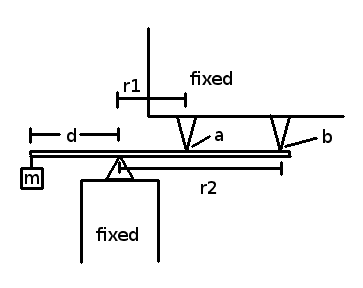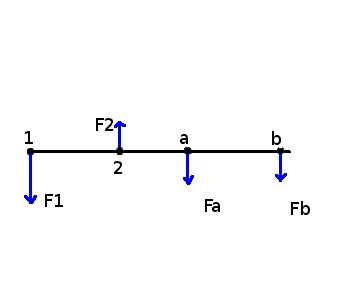# How is the torque shared between two points?

Figure:1. Homework Statement

We know system is in equilibrium and we are given the mass m, and the distances d, r1, and r2.
We assume that the rotational inertia of the bar is negligible.

What are the forces at points a and b?

## Homework Equations

Torque = radius × Force
Force = mass * acceleration
ΣFi = 0
ΣTi =0
where i = {x,y,z}

## The Attempt at a Solution

The force of the mass is F=mg, therefore the torque produced is T = d×(mg) = d*mg*sin(pi / 2) = dmg. (Where g is 9.81 m/s^2.)

Since we are in equilibrium we know r1*Fa+r2*Fb=T. Where Fa and Fb are the forces on the bar at the points a and b respectively.

Now how is the opposing torque shared between the two points?
Perhaps the torques r1*Fa and r2*Fb are equal, or perhaps Fa and Fb are equal?

## Answers and Replies

Filip Larsen
Gold Member
Welcome to PF!

In equilibrium you know that the total torque around any point is zero. You can use that to your advantage here to directly get an equation for Fa and Fb.

Alternatively, you can use the equation for torque around the left support point as you wrote it and combine it with the equation for translational equilibrium.

Welcome to PF!

In equilibrium you know that the total torque around any point is zero. You can use that to your advantage here to directly get an equation for Fa and Fb.

Alternatively, you can use the equation for torque around the left support point as you wrote it and combine it with the equation for translational equilibrium.

Oh right, fantastic! Let me give that a try.

In equilibrium you know that the total torque around any point is zero. You can use that to your advantage here to directly get an equation for Fa and Fb.

Been working on it for a few hours and I'm quite stuck.
I'm trying the first method.
The following is what I think the free body diagram is.From which I get the following equations for the torque around each point
Torque around midpoint between 2 and a: (d + 0.5*r1)F1 - 0.5*r1F2 - 0.5*r1Fa - (0.5*r1 + r2)Fb = 0
Torque around 2: dF1 - r1Fa - r2Fb = 0
Torque around 1: dF2 - (d + r1)Fa - (d + r2)Fb = 0
Torque around a: (d + r1)F1 - r1F2 - (r2 - r1)Fb = 0
Torque around b: (d + r2)F1 - r2F2 + (r2 - r1)Fa = 0

However the matrix formed with all the combinations I have tried are singular.
Where am I going wrong?

This is for an independent project I'm doing rather than a course, is this in the right section?
If not where do I got to get direct help?
Can you show me how to solve this?

Filip Larsen
Gold Member
My apologies for giving you a wrong late night knee-jerk response for what I assumed to be a textbook problem. I should of course have analysed it better before answering - you even provided an excellent diagram. My bad for giving a false impression that this was easy to solve.

You are correct about your system of equations being singular. The problem you have stated is statically indeterminate  and thus have no single solution for Fa and Fb. To make your system determinate you will either have to remove a constraint (like a or b), or, if you are up to more advanced beam mechanics, expand the model to include a bending moment from the beam itself in which case you may want to skim some of the search results in  or get a textbook on beam mechanics.

 http://en.wikipedia.org/wiki/Statically_indeterminate
 http://www.google.com/search?q=statically+indeterminate+beams

My apologies for giving you a wrong late night knee-jerk response for what I assumed to be a textbook problem. I should of course have analysed it better before answering - you even provided an excellent diagram. My bad for giving a false impression that this was easy to solve.

You are correct about your system of equations being singular. The problem you have stated is statically indeterminate  and thus have no single solution for Fa and Fb. To make your system determinate you will either have to remove a constraint (like a or b), or, if you are up to more advanced beam mechanics, expand the model to include a bending moment from the beam itself in which case you may want to skim some of the search results in  or get a textbook on beam mechanics.

 http://en.wikipedia.org/wiki/Statically_indeterminate
 http://www.google.com/search?q=statically+indeterminate+beams

No worries! That seems to be everyone's first impression, including mine.

Thank you for the help! The more advanced beam mechanics is exactly what I'm looking for, do you recommend any books in particular?